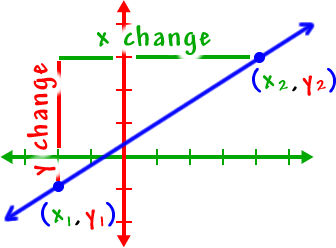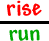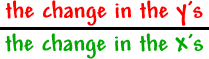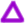Let's look at this in a way that will get us that formula for crunching slopes from two points:Look at theasThat is what gets us that familiar formula:In math and science, we often use a Greek capital "D"... delta ()...  to represent a "change".A Calculus notation you'll see a LOT ditches the Greek letter part and uses "d's"...It's a simple thing from Algebra, but the slopes of lines are going to be REALLY important in Calculus.